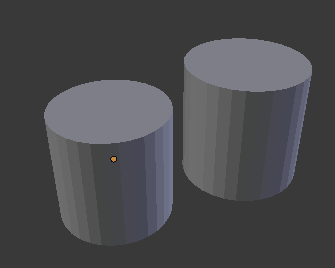# ray_cast bug in Blender 2.77+

I just found out there is a real bug in ray_cast.
Blender updated ray_cast in version 2.77, where it starts to return 4 values instead of 3. Since then there is a bug it seems. I am checking whether 2 objects interfere with coDEmanX’s great script from here: http://blender.stackexchange.com/questions/9073/how-to-check-if-two-meshes-intersect-in-python

It works very nicely until Blender 2.76. I wanted to port our code to 2.78 and detected the intersection-checking code does not work anymore. ray_cast is causing the trouble.I am using:

``````
obj1 = bpy.data.objects['Cylinder.6403']

obj2 = bpy.data.objects['Cylinder']

intersect = bmesh_check_intersect_objects(obj1, obj2)

if(intersect != 0):
print(obj1, ' intersects ', obj2)

``````

on the 2 geos in the attached ray_cast_error.blend. When I move the geos around, I sometimes get correct intereference results, and sometimes not. If you test with the default positions in the file, and Blender 2.77+, then the result is incorrect (it says the 2 geos collide, where in fact they are not).

Here I call ray_cast with 3 / 4 return values depending on the Blender version:

``````<b>  if ((bpy.app.version == 2) and (bpy.app.version &lt; 77)):

#Return (location, normal, index):
location, normal, index = ray_cast(co_1, co_2)
else:
#Return (result, location, normal, index):
result, location, normal, index = ray_cast(co_1, co_2)

</b>
``````

ray_cast_error.blend (481 KB)

Attached

New object and scene raycast functions also takes “origin vector” and “direction normal” as inputs.
Not “start” and “end” vectors like old versions.

``````<b>ray_cast(co_1, co_2 - co_1)  </b># second vector to normal (assume mathutils.Vector)
``````

Thank you!!

Wow, I just tried it, in Blender 2.78, and it does not work???

This is the “check_intersect” code I am using:

``````
<b>def bmesh_check_intersect_objects(obj, obj2):
#Check if any faces intersect with the other object
#
myresult = -1
#  returns a boolean
#
assert(obj != obj2)
#
# Triangulate
bm = bmesh_copy_from_object(obj, transform=True, triangulate=True)
bm2 = bmesh_copy_from_object(obj2, transform=True, triangulate=True)
#
# If bm has more edges, use bm2 instead for looping over its edges
# (so we cast less rays from the simpler object to the more complex object)
#if len(bm.edges) &gt; len(bm2.edges):
#bm2, bm = bm, bm2
#
# Create a real mesh (lame!)
scene = bpy.context.scene
me_tmp = bpy.data.meshes.new(name="~temp~")
bm2.to_mesh(me_tmp)
bm2.free()
obj_tmp = bpy.data.objects.new(name=me_tmp.name, object_data=me_tmp)
scene.update()
ray_cast = obj_tmp.ray_cast
#
intersect = False
#
EPS_NORMAL = 0.000001
EPS_CENTER = 0.01  # should always be bigger
#
#for ed in me_tmp.edges:
for ed in bm.edges:
v1, v2 = ed.verts
#
# setup the edge with an offset
co_1 = v1.co.copy()
co_2 = v2.co.copy()
co_mid = (co_1 + co_2) * 0.5
no_mid = (v1.normal + v2.normal).normalized() * EPS_NORMAL
co_1 = co_1.lerp(co_mid, EPS_CENTER) + no_mid
co_2 = co_2.lerp(co_mid, EPS_CENTER) + no_mid
#
#
if ((bpy.app.version == 2) and (bpy.app.version &lt; 77)):
#Return (location, normal, index):
location, normal, index = ray_cast(co_1, co_2)
if index != -1:
myresult = 1
else:
#Return (result, location, normal, index):
#not yet tested, but should be ok:
result = ray_cast(co_1, co_2 - co_1)
# second vector to normal (assume mathutils.Vector)
#2.78: result, location, normal, index, object, matrix = ray_cast(<i>origin</i>, <i>direction</i>, <i>distance=1.70141e+38</i>)
print (result)
print (location)
print (index)
if result != -1:
myresult = 1
#
if myresult != -1:
intersect = True
break
#
bpy.data.objects.remove(obj_tmp)
bpy.data.meshes.remove(me_tmp)
#
scene.update()
#
return intersect
#END def
</b>

``````

Here different way to do this:

``````import bpy
import bmesh
from mathutils.bvhtree import BVHTree

b = bpy.data.objects

bm1, bm2 = bmesh.new(), bmesh.new()

bm1.from_mesh(b.data)
bm2.from_mesh(b.data)

bmesh.ops.transform(bm1, matrix=b.matrix_world, space=b.matrix_world, verts=bm1.verts)
bmesh.ops.transform(bm2, matrix=b.matrix_world, space=b.matrix_world, verts=bm2.verts)

T1 = BVHTree.FromBMesh(bm1)
T2 = BVHTree.FromBMesh(bm2)
if T1.overlap(T2):
out = True
else:
out = False

print(out)
``````

Just change b and b to your objects.

WOW, that’s working, MANY THANKS!!! You are great.

And it’s many times faster than the previous method